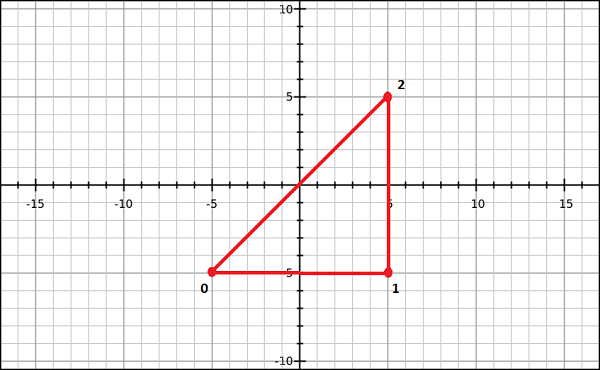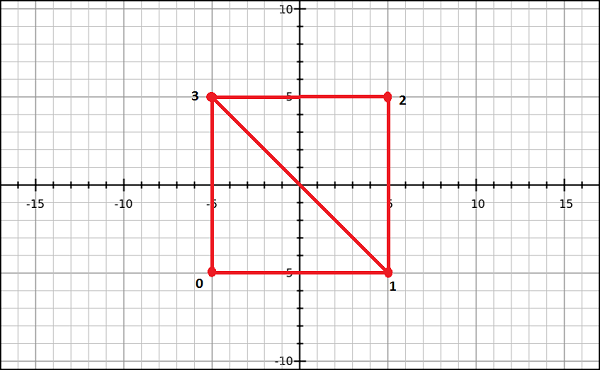# WebGL - Geometry

After obtaining the WebGL context, you have to define the geometry for the primitive (object you want to draw) and store it. In WebGL, we define the details of a geometry – for example, vertices, indices, color of the primitive – using JavaScript arrays. To pass these details to the shader programs, we have to create the buffer objects and store (attach) the JavaScript arrays containing the data in the respective buffers.

Note: Later, these buffer objects will be associated with the attributes of the shader program (vertex shader).

## Defining the Required Geometry

A 2D or 3D model drawn using vertices is called a mesh. Each facet in a mesh is called a polygon and a polygon is made of 3 or more vertices.

To draw models in the WebGL rendering context, you have to define the vertices and indices using JavaScript arrays. For example, if we want to create a triangle which lies on the coordinates {(5,5), (-5,5), (-5,-5)} as shown in the diagram, then you can create an array for the vertices as −

```var vertices = [
0.5,0.5,    //Vertex 1
0.5,-0.5,   //Vertex 2
-0.5,-0.5,  //Vertex 3
];
```Similarly, you can create an array for the indices. Indices for the above triangle indices will be [0, 1, 2] and can be defined as −

```var indices = [ 0,1,2 ]
```

For a better understanding of indices, consider more complex models like square. We can represent a square as a set of two triangles. If (0,3,1) and (3,1,2) are the two triangles using which we intend to draw a square, then the indices will be defined as −

```var indices = [0,3,1,3,1,2];
```Note

For drawing primitives, WebGL provides the following two methods −

• drawArrays() − While using this method, we pass the vertices of the primitive using JavaScript arrays.

• drawElements() − While using this method, we pass both vertices and indices of the primitive using JavaScript array.

## Buffer Objects

A buffer object is a mechanism provided by WebGL that indicates a memory area allocated in the system. In these buffer objects, you can store data of the model you want to draw, corresponding to vertices, indices, color, etc.

Using these buffer objects, you can pass multiple data to the shader program (vertex shader) through one of its attribute variables. Since these buffer objects reside in the GPU memory, they can be rendered directly, which in turn improves the performance.

To process geometry, there are two types of buffer objects. They are −

• Vertex buffer object (VBO) − It holds the per-vertex data of the graphical model that is going to be rendered. We use vertex buffer objects in WebGL to store and process the data regarding vertices such as vertex coordinates, normals, colors, and texture coordinates.

• Index buffer objects (IBO) − It holds the indices (index data) of the graphical model that is going to be rendered.

After defining the required geometry and storing them in JavaScript arrays, you need to pass these arrays to the buffer objects, from where the data will be passed to the shader programs. The following steps are to be followed to store data in the buffers.

• Create an empty buffer.

• Bind an appropriate array object to the empty buffer.

• Pass the data (vertices/indices) to the buffer using one of the typed arrays.

• Unbind the buffer (Optional).

## Creating a Buffer

To create an empty buffer object, WebGL provides a method called createBuffer(). This method returns a newly created buffer object, if the creation was successful; else it returns a null value in case of failure.

WebGL operates as a state machine. Once a buffer is created, any subsequent buffer operation will be executed on the current buffer until we unbound it. Use the following code to create a buffer −

```var vertex_buffer = gl.createBuffer();
```

Notegl is the reference variable to the current WebGL context.

## Bind the Buffer

After creating an empty buffer object, you need to bind an appropriate array buffer (target) to it. WebGL provides a method called bindBuffer() for this purpose.

### Syntax

The syntax of bindBuffer() method is as follows −

```void bindBuffer (enum target, Object buffer)
```

This method accepts two parameters and they are discussed below.

target − The first variable is an enum value representing the type of the buffer we want to bind to the empty buffer. You have two predefined enum values as options for this parameter. They are −

• ARRAY_BUFFER which represents vertex data.

• ELEMENT_ARRAY_BUFFER which represents index data.

Object buffer − The second one is the reference variable to the buffer object created in the previous step. The reference variable can be of a vertex buffer object or of an index buffer object.

### Example

The following code snippet shows how to use the bindBuffer() method.

```//vertex buffer
var vertex_buffer = gl.createBuffer();
gl.bindBuffer(gl.ARRAY_BUFFER, vertex_buffer);

//Index buffer
var Index_Buffer = gl.createBuffer();
gl.bindBuffer(gl.ELEMENT_ARRAY_BUFFER, index_buffer);
```

## Passing Data into the Buffer

The next step is to pass the data (vertices/indices) to the buffer. Till now data is in the form of an array and before passing it to the buffer, we need to wrap it in one of the WebGL typed arrays. WebGL provides a method named bufferData() for this purpose.

### Syntax

The syntax of bufferData() method is as follows −

```void bufferData (enum target, Object data, enum usage)
```

This method accepts three parameters and they are discussed below −

target − The first parameter is an enum value representing the type of the array buffer we used.The options for this parameter are −

• ARRAY_BUFFER which represents vertex data.

• ELEMENT_ARRAY_BUFFER which represents index data.

Object data − The second parameter is the object value that contains the data to be written to the buffer object. Here we have to pass the data using typed arrays.

Usage − The third parameter of this method is an enum variable that specifies how to use the buffer object data (stored data) to draw shapes. There are three options for this parameter as listed below.

• gl.STATIC_DRAW − Data will be specified once and used many times.

• gl.STREAM_DRAW − Data will be specified once and used a few times.

• gl.DYNAMIC_DRAW − Data will be specified repeatedly and used many times.

### Example

The following code snippet shows how to use the bufferData() method. Assume vertices and indices are the arrays holding the vertex and index data respectively.

```//vertex buffer
gl.bufferData(gl.ARRAY_BUFFER, new Float32Array(vertices), gl.STATIC_DRAW);

//Index buffer
gl.bufferData(gl.ELEMENT_ARRAY_BUFFER, new Uint16Array(indices), gl.STATIC_DRAW);
```

## Typed Arrays

WebGL provides a special type of array called typed arrays to transfer the data elements such as index vertex and texture. These typed arrays store large quantities of data and process them in native binary format which results in better performance. The typed arrays used by WebGL are Int8Array, Uint8Array, Int16Array, Uint16Array, Int32Array, UInt32Array, Float32Array, and Float64Array.

Note

• Generally, for storing vertex data, we use Float32Array; and to store index data, we use Uint16Array.

• You can create typed arrays just like JavaScript arrays using new keyword.

## Unbind the Buffers

It is recommended that you unbind the buffers after using them. It can be done by passing a null value in place of the buffer object, as shown below.

```gl.bindBuffer(gl.ELEMENT_ARRAY_BUFFER, null);
```

WebGL provides the following methods to perform buffer operations −

Sr.No. Methods and Description
1

void bindBuffer (enum target, Object buffer)

target − ARRAY_BUFFER, ELEMENT_ARRAY_BUFFER

2

void bufferData(enum target, long size, enum usage)

target − ARRAY_BUFFER, ELEMENT_ARRAY_BUFFER

usage − STATIC_DRAW, STREAM_DRAW, DYNAMIC_DRAW

3

void bufferData (enum target, Object data, enum usage)

target and usage − Same as for bufferData above

4

void bufferSubData(enum target, long offset, Object data)

target − ARRAY_BUFFER, ELEMENT_ARRAY_BUFFER

5 Object createBuffer()
6 void deleteBuffer(Object buffer)
7

any getBufferParameter(enum target, enum pname)

target − ARRAY_BUFFER, ELEMENT_ ARRAY_BUFFER

pname − BUFFER_SIZE, BUFFER_USAGE

8 bool isBuffer(Object buffer)